• Save

# Decimal Fraction Definition with Types, Formulas, Solutions and Examples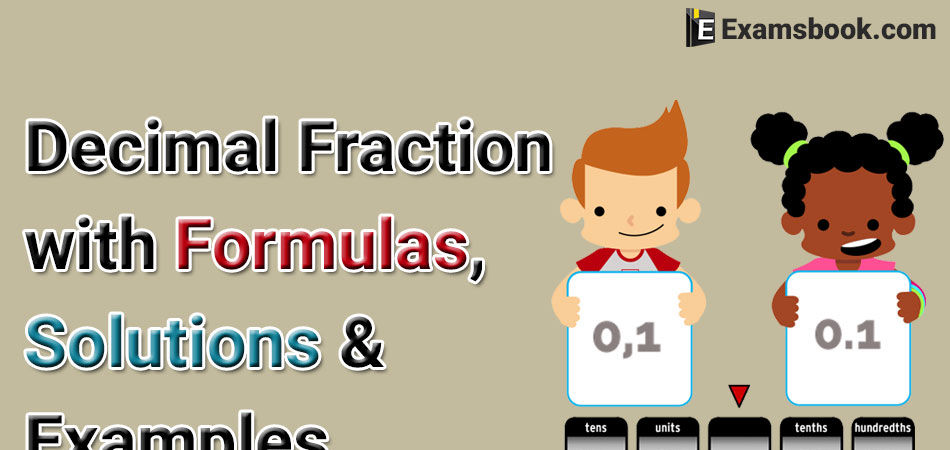Students often face difficulties while solving decimal fractions questions in the competitive exams because they get confused to using formulas. So here in this blog, you can easily know about decimal fraction definition, decimal fraction formulas, decimal fraction solutions with examples.

You should understand the decimal fraction topic by these important steps to improve your performance in the competitive exams.

## Complete detail of Decimal Fraction

Decimal fractions : Fraction in which denominator are powers of 10 are known as decimal fraction.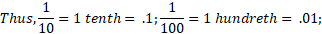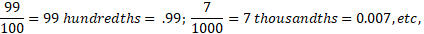Conversion of a Decimal Into Vulgar Fraction : Put 1 in the denominator under the decimal point and annex with it as many zero as is the number of digits after the decimal point. Now, remove the decimal point and reduce the fraction to its lowest terms.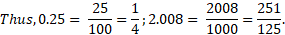### Operation on Decimal Fraction

1. Addition and subtraction of decimal fraction

2. Multiplication of Two or More Decimal Fraction

3. Dividing a Decimal Fraction by an integer

4. Division of Decimal fractions

#### 1. Addition and subtraction of decimal fraction:

For addition or subtraction of decimal fractions, write the decimal fraction in such a way that all the decimal points are in the align form, then these numbers can add or subtract in simple manner.

Ex. 353.5 + 2.32 + 43.23 is equal to

(A) 400.00

(B) 398.5

(C) 399.05

(D) None of these

Ans : C

Solution:

353.50 + 2.32 + 43.23 = 399.05

#### 2. Multiplication of Two or More Decimal Fraction:

For multiplication of two or more decimal fractions, multiply then without considering the decimal points and then in the product, decimal point is marked as many places of decimal as the sum of the numbers of decimal in the given number.

Ex. 4.3 × 0.13 is equal to

(A) 0.599

(B) 0.622

(C) 5.99

(D) None of these

Ans: A

Solution:

43 × 13 = 599

Sum of the decimal places = (1+2) = 3

⸫ Required products = 0.599

#### 3. Dividing a Decimal Fraction by an integer:

If we divide a decimal number by an integer, then at first divide the number ignoring the decimal point and at last put the decimal after the number of digits (from right) according at in the given number.

Ex. Divide 0.81 by 9 is equal to

(A) 0.9

(B) 0.009

(C) 9

(D) None of these

Ans : B

Solution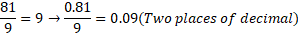If we divide the decimal number by multiple of 10. Then we move the decimal point to the left by the respective number of zeroes.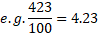#### 4. Division of Decimal Fraction:

If divisor and dividend both are decimal numbers, then first convert then into then simple fraction by putting number of zeroes in the denominators of both. Now, divide them.

Ex. Divide 0.42 by 0.007 is equal to

(A) 600

(B) 60

(C) 6000

(D) None of these

Ans: B

Solution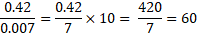Recurring Decimals: The decimal fractions, in which one or more decimal digits are repeated again and again, are called recurring decimal fractions. E.g.,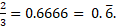Here, a line is drawn on the digits which are repeated.

Pure and Mixed Recurring Decimal: In a recurring decimal, if all the figures are repeated after the decimal point, then it is called a pure recurring decimal and if some figures are not repeated, while some of them are repeated, then it is called a mixed recurring decimal.

To convert a Mixed Recurring Decimal into a simple fraction: Write the repeated figures only once in the numerator and place as many nines in the denominator as the number of figures repeating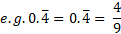To convert a Mixed Recurring Decimal into a simple fraction: Write the numerator take the difference between the numbers formed by all the digits after decimal point (repeated digits will be taken only once) and the number formed by nor-repeating digits.

In the denominator place as many nine as there are repeating digits and after nine put as many zeroes as the number of non-repeating digits.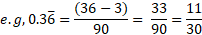To know more, visit on the next page